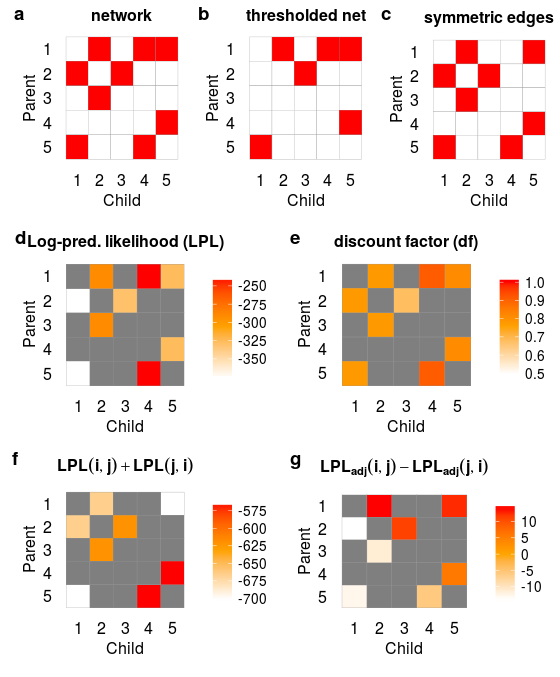multdyn: Multiregression Dynamic Models for directed dynamic functional brain network analysis.

The aim of this package is to study directed functional connectivity in fMRI. Multiregression Dynamic Models (MDM) belong to the family of Dynamic Bayesian Networks. MDM searches through all possible parent nodes for a specific node and provides an interpretable fit in terms of regression model for each network node (Costa et al., 2015).

Current research aims to fully characterize MDM using simulations and big data from the Human Connectome Project (HCP) in order to test validity and different aspects of reliability (test-retest reliability, out-of-sample reliability) in order to make this new method available for neuroimaging.

1. Costa, L., Smith, J., Nichols, T., Cussens, J., Duff, E. P., and Makin, T. R. (2015). Searching Multiregression Dynamic Models of resting-state fMRI networks using integer programming. Bayesian Analysis, 10(2), 441–478. doi:10.1214/14-BA913.
2. Smith, S. M., Miller, K. L., Salimi-Khorshidi, G., Webster, M., Beckmann, C. F., Nichols, T. E., et al. (2011). Network modelling methods for FMRI. NeuroImage, 54(2), 875–91. doi:10.1016/j.neuroimage.2010.08.063.

Reference this package

Schwab, S., Harbord, R., Costa, L., Nichols, T.E. (2017). multdyn: A package for Multiregression Dynamic Models (MDM). Available at: https://github.com/schw4b/multdyn.

User Guide

Installation

The installation with dependencies is approx. 86MB.

From CRAN:

``install.packages("multdyn")``

``````install.packages("devtools")
library(devtools)
install_github("schw4b/multdyn")``````

Latest develop version

``````install.packages("devtools")
library(devtools)
install_github("schw4b/multdyn", ref = "develop")``````

Running a MDM example with simulated data

We load simulation data from Smith et al. (2011) of a 5-node network with 200 samples (time points) of one subject. Time series should be mean centered.

``````library(multdyn)
data("utestdata")
dim(myts)
 200   5``````

Now, let’s do a full search across all possible parent models of n2(n-1). Here, with n=5, we have 16 possible models for each node.

``````result=exhaustive.search(myts,3)
result\$model.store
[,1]      [,2]      [,3]      [,4]      [,5]      [,6]     [,7]      [,8]      [,9]     [,10]     [,11]     [,12]     [,13]     [,14]     [,15]     [,16]
[1,]    1.0000    2.0000    3.0000    4.0000    5.0000    6.0000    7.000    8.0000    9.0000   10.0000   11.0000   12.0000   13.0000   14.0000   15.0000   16.0000
[2,]    0.0000    1.0000    2.0000    4.0000    5.0000    1.0000    1.000    1.0000    2.0000    2.0000    4.0000    1.0000    1.0000    1.0000    2.0000    1.0000
[3,]    0.0000    0.0000    0.0000    0.0000    0.0000    2.0000    4.000    5.0000    4.0000    5.0000    5.0000    2.0000    2.0000    4.0000    4.0000    2.0000
[4,]    0.0000    0.0000    0.0000    0.0000    0.0000    0.0000    0.000    0.0000    0.0000    0.0000    0.0000    4.0000    5.0000    5.0000    5.0000    4.0000
[5,]    0.0000    0.0000    0.0000    0.0000    0.0000    0.0000    0.000    0.0000    0.0000    0.0000    0.0000    0.0000    0.0000    0.0000    0.0000    5.0000
[6,] -353.6734 -348.1476 -329.9119 -359.3472 -346.9735 -348.4044 -355.495 -347.4541 -337.3578 -333.6073 -353.8772 -349.6878 -346.9843 -358.2056 -341.7462 -355.5821
[7,]    0.5000    0.7300    0.6800    0.7700    0.7600    0.7800    0.800    0.7900    0.7300    0.7700    0.8100    0.7900    0.8300    0.8400    0.7800    0.8300``````

The table colums are the 16 different models. First row indicates model number, rows 2-5 the parents, row 6 the log predictive likelihood (LPL), and row 7 the discount factor (delta). To get the winning model, we simply maximaze across LPLs.

``````which.max(result\$model.store[6,])
 3``````

Model number 3 with node 2 as a parent is most likely.

Analysis on the subject level

We do a full search on the subject level (exhautive search on each node). The list returned contains all the models (models), the winning models (winner), the adjacency matrix of the network (adj), and a thresholded network (thr). The thresholed network is a reduced version of the network, favorizing the simpler model by comparing the Bayes factors. If the Bayes factor between the models (symetric edge vs. asymetric edge) is similar, the simpler asymetric model is favorized.

``````s=subject(myts)
names(s)

The adj structure contains the adjacency matrix of the network (am, Fig. 1a), the LPLs (lpl, Fig. 1d), and the discount factors (df, Fig. 1e). The thr structure contains a matrix of edges that are bidirectional/symmetric (bi, Fig. 1c), the two matrices of LPLs with the first containing LPLs for bidirectional edges (Fig. 1f), the second contains adjusted LPLs (Fig. 1g) for asymetric models (after removing one or the other of the symetric edges, and the thresholded adjacency matrix (am, Fig 1b).

``````names(s\$adj)
 "am"  "lpl" "df"
names(s\$thr)
 "bi"   "lpls" "am"``````

The full network and a thresholded network can be plotted as follows

``````p1 = gplotMat(s\$adj\$am, hasColMap = F, title = "network")
p2 = gplotMat(s\$thr\$am, hasColMap = F, title = "thresholded net")
p3 = gplotMat(s\$thr\$bi, hasColMap = F, title = "symmetric edges")

p4 = gplotMat(s\$adj\$lpl, title = "Log-pred. likelihood (LPL)",
p5 = gplotMat(s\$adj\$df, title = "discount factor (df)", lim = c(0.5, 1))
p6 = gplotMat(s\$thr\$lpls[, , 1], title = expression(bold(LPL(i,j) +
LPL(j,i))), lim = c(min(s\$thr\$lpls[, , 1], na.rm = T), max(s\$thr\$lpls[, , 1], na.rm = T)))

difference = s\$thr\$lpls[, , 2] - t(s\$thr\$lpls[, , 2])
p7 = gplotMat(difference, title = expression(bold(LPL[adj](i,j) - LPL[adj](j,i))), lim = c(min(difference, na.rm = T), max(difference, na.rm = T)))

top = plot_grid(p1, p2, p3, ncol = 3, labels = c("a", "b", "c"))
bot = plot_grid(p4, p5, p6, p7, ncol = 2, labels = c("d", "e", "f", "g"))
plot_grid(top, bot, ncol = 1, rel_heights = c(0.5, 1))``````Figure 1

HPC guide (high performance computing)

Timing estmates

Estimates for a time-series with 1200 samples (HCP), and for a 2.8GHz CPU.

No. of nodes Time
3 0.2 sec
4 0.5 sec
5 1 sec
6 2.2 sec
7 4.8 sec
8 10 sec
9 22 sec
10 48 sec
11 1 min 46 sec
12 3 min 44 sec
13 8 min 8 sec
15 38 min
20 30 hours
25 58 days

Timings are for one node only. To estimate the full network (all parents of all the nodes, the numbers above have to be multiplied by the number of nodes (e.g., a 8-node network takes 80 sec.)

Install or update MDM on Buster super-computer (buster.stats.warwick.ac.uk)

From bash terminal on buster run:

``````\$ module load git
``````install.packages("devtools") # run only once